# Bredon cohomology

An ordinary equivariant cohomology for a finite group, defined in [a1], on the category-CW of-complexes (cf. Complex; CW-complex). The objects of-CW are the CW-complexeswith a cellular action of, satisfying the condition that, for every subgroup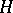of, the fixed point set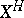is a subcomplex of. The morphisms are the cellular-mappings. Let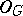be the full subcategory of-CW whose objects are the-orbits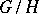, whereis a subgroup of. For every contravariant functorfromto the category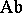of Abelian groups, there exists a Bredon cohomology theorywhich, after restriction to, vanishes forand is equal tofor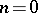.

Letbe the chain complex of functors fromtosuch that, for every subgroupof,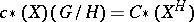is the ordinary cellular chain complex of. Then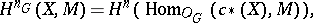where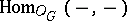denotes the set of natural transformations of functors. The functors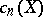are projective objects in the category of coefficient systems and there is a spectral sequence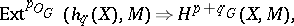where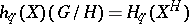.

Letbe a-space with base point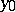(cf., e.g., Equivariant cohomology). Important examples of coefficient systems are the homotopy group functorsdefined by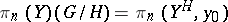. The obstruction theory for-mappingsis formulated in terms of the cohomology groups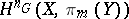. For any coefficient systemand natural number, there is a pointed Eilenberg–MacLane-complex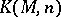such that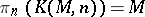and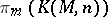vanishes whenever. For every-complex,, where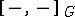denotes-homotopy classes of-mappings.

If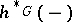is an equivariant cohomology theory defined on the category-CW, then there exists an Atiyah–Hirzebruch-type spectral sequence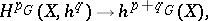where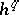is the restriction of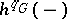to. Bredon cohomology for an arbitrary topological group is studied in [a4] and [a5]. Singular ordinary equivariant cohomology is defined in [a2] (the finite case) and in [a3]. If a coefficient systemis a Mackey functor, then the Bredon cohomology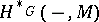can be extended to an ordinary-graded cohomology [a6].